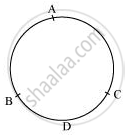# Write True Or False. Give Reasons for Your Answers. If a Circle is Divided into Three Equal Arcs, Each is a Major Arc. - Mathematics

MCQ
True or False

If a circle is divided into three equal arcs, each is a major arc.

• True

• False

#### Solution

False.

Consider three arcs of same length as AB, BC, and CA. It can be observed that for minor arc BDC, CAB is a major arc. Therefore, AB, BC, and CA are minor arcs of the circle.Concept: Concept of Circle - Centre, Radius, Diameter, Arc, Sector, Chord, Segment, Semicircle, Circumference, Interior and Exterior, Concentric Circles
Is there an error in this question or solution?
Chapter 10: Circles - Exercise 10.1 [Page 171]

#### APPEARS IN

NCERT Class 9 Maths
Chapter 10 Circles
Exercise 10.1 | Q 2.3 | Page 171
Share